# RS Aggarwal Class 7 Solutions Chapter 4 Rational Numbers Ex 4A

## RS Aggarwal Class 7 Solutions Chapter 4 Rational Numbers Ex 4A

These Solutions are part of RS Aggarwal Solutions Class 7. Here we have given RS Aggarwal Solutions Class 7 Chapter 4 Rational Numbers Ex 4A.

Other Exercises

Question 1.
Solution:
(i) Rational numbers: The numbers of the form $$\frac { p }{ q }$$ where p and q are integers and q ≠ 0, are called rational numbers.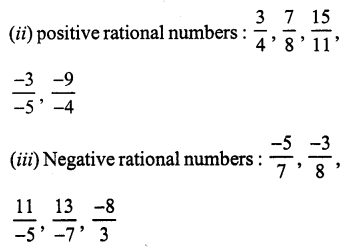(iv) Yes, there is one rational number (0) which is neither positive nor negative.

Question 2.
Solution: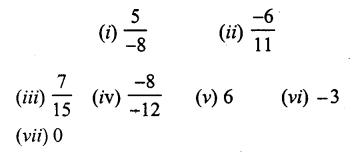(viii) $$\frac { 0 }{ 1 }$$ are all rational number but $$\frac { 1 }{ 0 }$$ and $$\frac { 0 }{ 0 }$$ are not rational number as their denominator is zero.

Question 3.
Solution:
(i) Numerator = 8, denominator =19
(ii) Numerator = 5, denominator = – 8
(iii) Numerator =-13, denominator =15
(iv) Numerator = – 8, denominator = -11
(v) Numerator = 9, denominator = 1

Question 4.
Solution: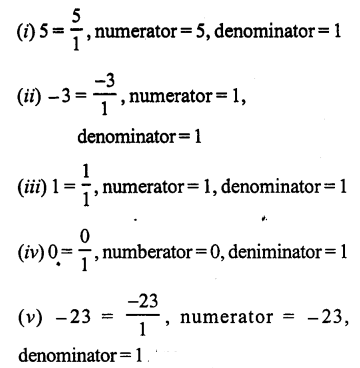Question 5.
Solution:
According to the definition, a rational number is positive if both of numerator and denominator have same signs. Therefore
(iii), (iv) and (vi) 8 are positive rational numbers.

Question 6.
Solution:
According to the definition, a rational number is negative if numerator and denominator have opposite sign. Therefore.
(iii), (iv), (v), (vi) are all negative rational numbers.

Question 7.
Solution:
Equivalent rational numbers of each are given below: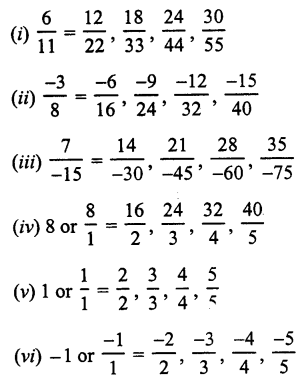Question 8.
Solution: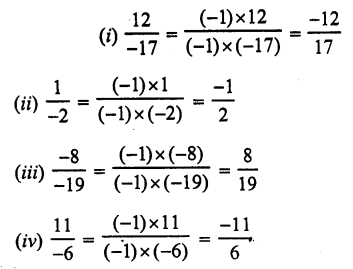Question 9.
Solution: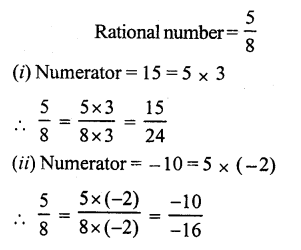Question 10.
Solution: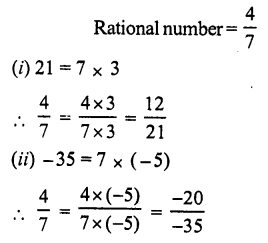Question 11.
Solution: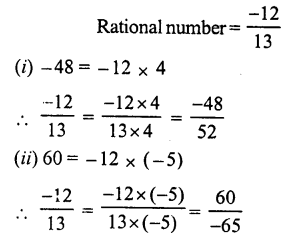Question 12.
Solution: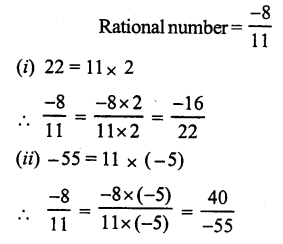Question 13.
Solution: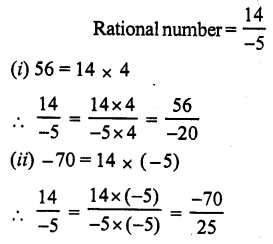Question 14.
Solution: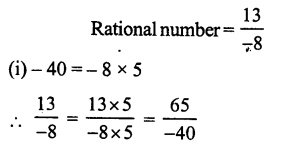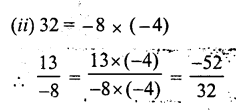Question 15.
Solution: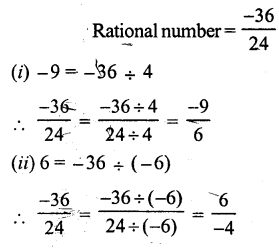Question 16.
Solution: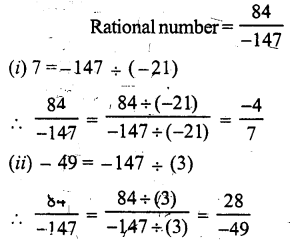Question 17.
Solution: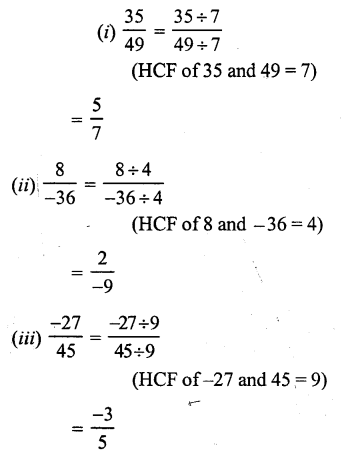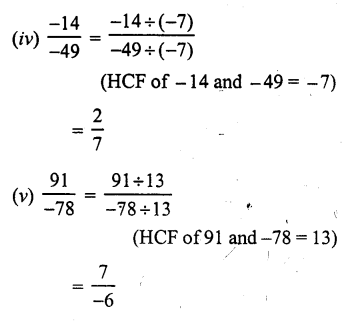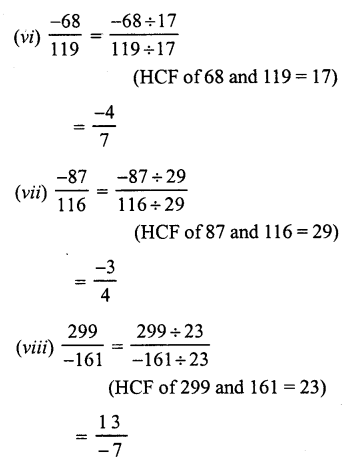Question 18.
Solution: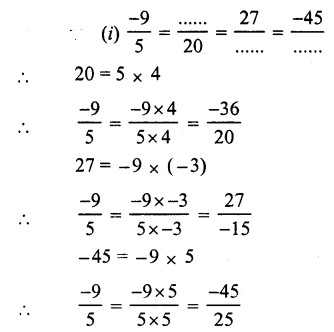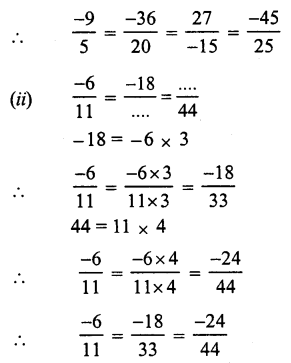Question 19.
Solution: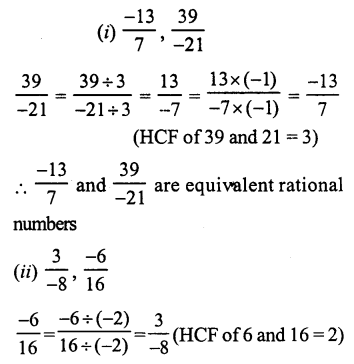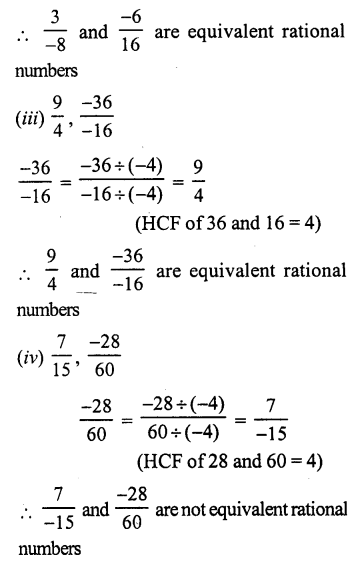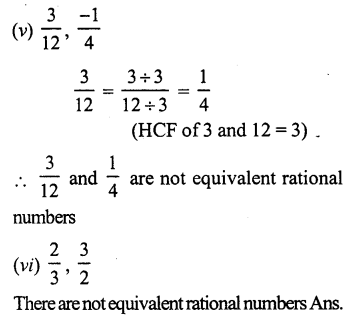Question 20.
Solution: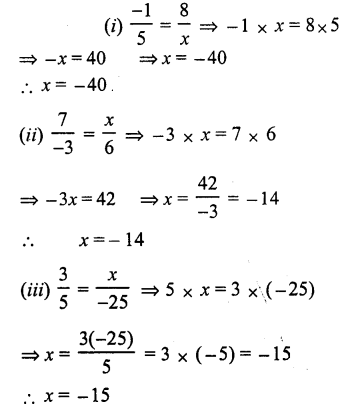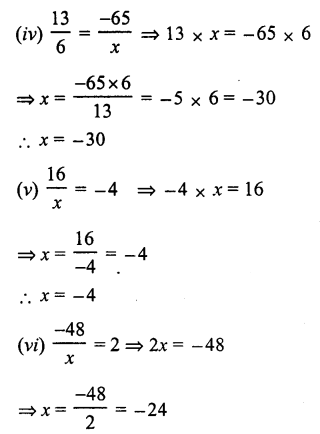Question 21.
Solution: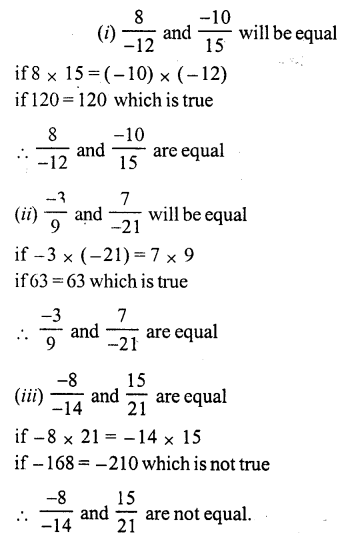Question 22.
Solution:
(i) False, as there is no end of smallest and largest rational number,
(ii) True.
(iii) False, as zero is a rational number but the division of zero is meaningless.
(iv) True.
(v) False, every rational is not a fraction
In a fraction, numerator and denominators is a whole number but the denominator can’t be zero

Hope given RS Aggarwal Solutions Class 7 Chapter 4 Rational Numbers Ex 4A are helpful to complete your math homework.

If you have any doubts, please comment below. Learn Insta try to provide online math tutoring for you.